# Rectangle

The length of one side of the rectangle is three times the length of the second side. What are the dimensions of the rectangle if its circumference 96 cm?

a =  36
b =  12

### Step-by-step explanation:

a=3b
2(a+b)=96

a=3•b
2•(a+b)=96

a-3b = 0
2a+2b = 96

a = 36
b = 12

Our linear equations calculator calculates it.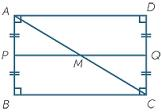Did you find an error or inaccuracy? Feel free to write us. Thank you!Tips to related online calculators
Do you have a linear equation or system of equations and looking for its solution? Or do you have a quadratic equation?
Do you want to convert length units?

#### You need to know the following knowledge to solve this word math problem:

We encourage you to watch this tutorial video on this math problem:

## Related math problems and questions:

• Second sideCalculate the length of the other side of the rectangle if its circumference is 60 cm and one side is 10 cm long.
• Triangle ABCIn a triangle ABC side b measure 10 cm less than the side a and side b is half of the side c. Calculate the length of sides if the circumference of the triangle is 42 cm.
• Table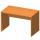Find the circumference of the table, where the long side is 1.28 meters and the short side is 86 cm.
• Tablecloth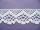The table top is 90 cm long and 50 cm wide. How many cm lace edging is necessary for the tablecloth that hangs on each side by 10 cm?
• GardensThe garden has the square shape with circumference 124 m. Divide it into two rectangular gardens, one should circumference 10 meters more than the second. What size will have a gardens?
• DiamondThe diamond has a circumference of 48cm. Calculate the length of its side in dm.
• MO-Z5-3-66 tilesThe picture shows a square tiles with side 10 dm which is composed of four identical small rectangles and squares. Circumference of small square is five times smaller than the circumference of the entire tile. Determine the dimensions of the rectangle.
• Do you solve this?Determine area S of rectangle and length of its sides if its perimeter is 102 cm.
• Ratio - rectangleThe rectangle has dimensions 6 cm and 9 cm. How many times increases area and its perimeter, when its dimensions increase in the ratio 5:3?
• RhombusFind the length of each side of rhombus if the perimeter is 89 cm long.
• Dimensions 3A perimeter of a rectangular field is 96 meters. The length is four meters less than three times the width. Find the length and width (its dimensions).
• Three elephants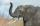Three elephants eat three piles of hay in 150 minutes. For how long does 96 elephants eat  96 stacks of hay?
• Fence of the garden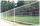The garden has dimensions of 5m and 400cm. How many meters meshes are needed for fencing the plot?
• Ratio of perimeters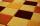Rectangle ABCD has dimensions 3 cm and 4 cm, KLMN rectangle has dimensions 4 cm and 12 cm. Calculate the ratio of the perimeter ABCD and perimeter KLMN.
• DivideDivide substance 110 cm long to two parts so that it first part is 10 cm longer than the second part and one part will be 10 times longer than the second portion. How long the parts will be?
• Perimeter from areaWhat is the perimeter of the square if its content is 64 cm2?
• Three rectangles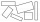Some wire is used to make 3 rectangles: A, B, and C. Rectangle B's dimensions are 3/5 cm larger than Rectangle A's dimensions, and Rectangle C's dimensions are again 3/5 cm larger than Rectangle B's dimensions. Rectangle A is 2 cm by 3 1/5 cm. What is the